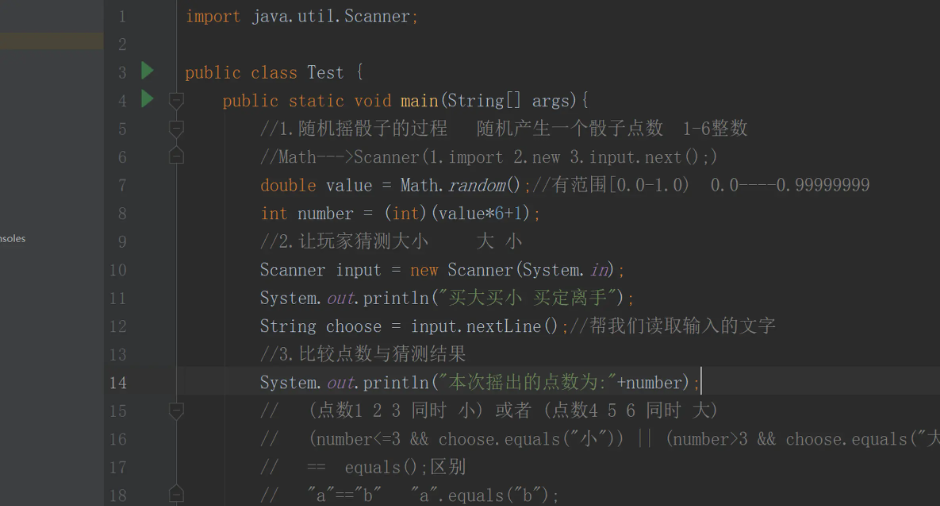# #冲刺创作新星#后端Java随机比大小游戏实战讲解 原创

0收藏## - 利用Math方法实现随机数

#### 1.首先用到的是print函数，对用户进行提醒进一步的操作

``````通过System.out.print();提示用户进行选择买大买小。
``````

#### 2.然后利用Scanner函数，对用户输出的数据进行一个抓取和储存

``````  使用Scanner函数调用方法，抓取用户输出的数据进行储存，储存格式为整型（int）类型

``````

#### 3.利用Math函数加载方法，实现随机数，进行判断定义结果

``````  使用Math方法函数进行随机判断，Math函数会随机给出一个数字范围为（0.0-1.0），然

``````
``````
public class Test {
public static void main(String[] args){
//1.随机摇骰子的过程   随机产生一个骰子点数  1-6整数
//Math--->Scanner(1.import 2.new 3.input.next();)
double value = Math.random();//有范围[0.0-1.0)  0.0----0.99999999
int number = (int)(value*6+1);
//2.让玩家猜测大小     大 小
Scanner input = new Scanner(System.in);
System.out.println("买大买小 买定离手");
String choose = input.nextLine();//帮我们读取输入的文字
//3.比较点数与猜测结果
System.out.println("本次摇出的点数为:"+number);
//   (点数1 2 3 同时 小) 或者 (点数4 5 6 同时 大)
//   (number<=3 && choose.equals("小")) || (number>3 && choose.equals("大"))
//   ==  equals();区别
//   "a"=="b"   "a".equals("b");
if((number<=3 && choose.equals("小")) || (number>3 && choose.equals("大"))){
System.out.println("恭喜您 猜对啦");
}else{
System.out.println("对不起 猜错啦 ");
}
}
}

``````

### 代码优先级规范

``````如果在编写程序时，对优先级有歧义，或者是对优先级了解不是很深，可以通过添加括号进行避免。
``````

### if语句规范

``````有经验的程序员往往在写if语句时，经常会看到if(NULL = p),这样做可以防止将if(p == NULL)写成
if(p = NULL),所以在写条件判断时，可以用括号这样隔开，防止写错。

``````帖子
视频
声望
粉丝
最近发布
热门推荐
相关问题
社区精华内容